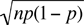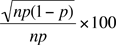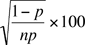Name: Glenn Level of question: University Who is asking: Forester/Biometrician Hi Math Central, What is the correct formula for coefficient of variation for a binomial distribution? Cheers, Glenn Hi Glenn, The coefficient of variation is the standard deviation divided by the mean, and then usually multiplied by 100 to express it as a percentage. It is thus the standard deviation expressed as a percentage of the mean. The mean of the binomial distribution with parameters n and p is np and the standard deviation isand thus the coefficient of variation iswhich simplifies toPenny